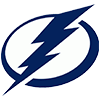DraftKings 2019 Eastern Conference Odds
+375
3.75 to 1Lightning
16.5% implied probability

+450
4.5 to 1Bruins
14.2% implied probability

+550
5.5 to 1Capitals
12.0% implied probability

+650
6.5 to 1Maple Leafs
10.4% implied probability

+700
7 to 1Hurricanes
9.8% implied probability

+700
7 to 1Penguins
9.8% implied probability

+900
9 to 1Islanders
7.8% implied probability

+1500
15 to 1Flyers
4.9% implied probability

+1800
18 to 1Panthers
4.1% implied probability

+2700
27 to 1Canadiens
2.8% implied probability

+3200
32 to 1Blue Jackets
2.4% implied probability

+3500
35 to 1Rangers
2.2% implied probability

+4500
45 to 1Sabres
1.7% implied probability

+10000
100 to 1Senators
0.8% implied probability

+12500
125 to 1Devils
0.6% implied probability

+75000
750 to 1Red Wings
0.1% implied probability